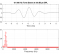## Matlab CWT ( Continuous Wavelet Transform ) Manual CalculationStarted by 3 years ago4 replieslatest reply 3 years ago330 views

Hello ,

I am wondering if anyone knows how to manually calculate the CWT step by step and be willing to show me ? Below is the CWT definition :

I am currently using Matlab to calculate the CWT scale matrix. However for my own design purposes I'd like to be able to manually calculate the CWT with some random data in Excel. I just want to see and understand the calculation that creates the CWT Matrix.

Thanks,

Richard

[ - ]Richard,

Please see my thesis on the fast wavelet transform.  It was a long time ago, but I think there is some Matlab and foundation in there that will help.

Regards,

Keith

[ - ]Keith,

Thanks for the link / info; I'll check it out.

Richard

[ - ]Richard,

I'd suggest stepping through the CWT function in Matlab using the debug options.  Since you are using this function now, understanding its functionality could be a more direct and efficient path to make the changes you want.

Michael.

[ - ]